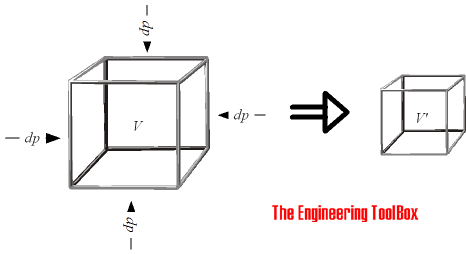Engineering ToolBox - Resources, Tools and Basic Information for Engineering and Design of Technical Applications!

# Bulk Modulus and Fluid Elasticity

## Introduction to - and definition of - Bulk Modulus Elasticity commonly used to characterize the compressibility of fluids.

The Bulk Modulus Elasticity - or Volume Modulus - is a material property characterizing the compressibility of a fluid - how easy a unit volume of a fluid can be changed when changing the pressure working upon it.The Bulk Modulus Elasticity can be calculated as

K = - dp / (dV / V0

= - (p1 - p0) / ((V1 - V0) / V0)                       (1)

where

K = Bulk Modulus of Elasticity (Pa, N/m2)

dp = differential change in pressure on the object (Pa, N/m2)

dV = differential change in volume of the object (m3)

V0 = initial volume of the object  (m3)

p0 = initial pressure (Pa, N/m2)

p1 = final pressure (Pa, N/m2)

V1 = final volume (m3)

The Bulk Modulus Elasticity can alternatively be expressed as

K = dp / (dρ / ρ0

= (p1 - p0) / ((ρ1 - ρ0) / ρ0)                              (2)

where

dρ = differential change in density of the object   (kg/m3)

ρ0 = initial density of the object  (kg/m3)

ρ1 = final density of the object (kg/m3)

An increase in the pressure will decrease the volume (1). A decrease in the volume will increase the density (2).

• The SI unit of the bulk modulus elasticity is N/m2 (Pa)
• The imperial (BG) unit is lbf/in2 (psi)
• 1 lbf/in2 (psi) = 6.894 103 N/m2 (Pa)

A large Bulk Modulus indicates a relative incompressible fluid.

Bulk Modulus for some common fluids:

FluidBulk Modulus
- K -
Imperial Units - BG
(105 psi, lbf/in2)
SI Units
(109 Pa, N/m2)
Acetone 1.34 0.92
Benzene 1.5 1.05
Carbon Tetrachloride 1.91 1.32
Ethyl Alcohol 1.54 1.06
Gasoline 1.9 1.3
Glycerin 6.31 4.35
ISO 32 mineral oil 2.6 1.8
Kerosene 1.9 1.3
Mercury 41.4 28.5
Paraffin Oil 2.41 1.66
Petrol 1.55 - 2.16 1.07 - 1.49
Phosphate ester 4.4 3
SAE 30 Oil 2.2 1.5
Seawater 3.39 2.34
Sulfuric Acid 4.3 3.0
Water (10 oC) 3.12 2.09
Water - glycol 5 3.4
Water in oil emulsion 3.3 2.3
• 1 GPa = 109 Pa (N/m2)

Stainless steel with Bulk Modulus 163 109 Pa is aprox. 80 times harder to compress than water with Bulk Modulus 2.15 109 Pa.

### Example - Density of Seawater in the Mariana Trench

- the deepest known point in the Earth's oceans - 10994 m.The hydrostatic pressure in the Mariana Trench can be calculated as

p1 = (1022 kg/m3) (9.81 m/s2) (10994 m)

= 110 106 Pa  (110 MPa)

The initial pressure at sea-level is 105 Pa and the density of seawater at sea level is 1022 kg/m3.

The density of seawater in the deep can be calculated by modifying (2) to

ρ1 = ((p1 - p0) ρ0  + K ρ0) / K

= (((110 106 Pa) - (1 105 Pa)) (1022 kg/m3) + (2.34 109 Pa) (1022 kg/m3)) / (2.34 109 Pa)

= 1070 kg/m3

Note! - since the density of the seawater varies with dept the pressure calculation could be done more accurate by calculating in dept intervals.

Temperature
(oC)
Bulk Modulus
(109 Pa)
0.01 1.96
10 2.09
20 2.18
30 2.23
40 2.26
50 2.26
60 2.25
70 2.21
80 2.17
90 2.11
100 2.04

## Related Topics

• ### Fluid Mechanics

The study of fluids - liquids and gases. Involving velocity, pressure, density and temperature as functions of space and time.
• ### Material Properties

Material properties of gases, fluids and solids - densities, specific heats, viscosities and more.

## Related Documents

• ### Cauchy Number

Introduction to the Cauchy Number.
• ### Heavy Water - Thermophysical Properties

Thermodynamic properties of heavy water (D2O) like density, melting temperature, boiling temperature, latent heat of fusion, latent heat of evaporation, critical temperature and more.
• ### Liquids - Densities vs. Pressure and Temperature Change

Densities and specific volume of liquids vs. pressure and temperature change.
• ### Mach Number

An introduction to the Mach Number.
• ### Metals and Alloys - Bulk Modulus Elasticity

The Bulk Modulus - resistance to uniform compression - for some common metals and alloys.
• ### Minerals - Densities

Densities of minerals.
• ### Modulus of Rigidity

Shear Modulus (Modulus of Rigidity) is the elasticity coefficient for shearing or torsion force.
• ### Speed of Sound Equations

Calculate the speed of sound (the sonic velocity) in gases, fluids or solids.
• ### Water Hammer

Rapidly closing or opening valves - or starting stopping pumps - may cause pressure transients in pipelines known as surge or water hammers.

## Engineering ToolBox - SketchUp Extension - Online 3D modeling!

Add standard and customized parametric components - like flange beams, lumbers, piping, stairs and more - to your Sketchup model with the Engineering ToolBox - SketchUp Extension - enabled for use with older versions of the amazing SketchUp Make and the newer "up to date" SketchUp Pro . Add the Engineering ToolBox extension to your SketchUp Make/Pro from the Extension Warehouse !

We don't collect information from our users. More about

## Citation

• The Engineering ToolBox (2004). Bulk Modulus and Fluid Elasticity. [online] Available at: https://www.engineeringtoolbox.com/bulk-modulus-elasticity-d_585.html [Accessed Day Month Year].

Modify the access date according your visit.

9.19.12